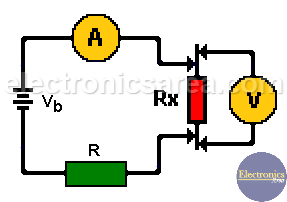# How to measure very low resistances?

There are special cases of a resistance measurement where the value of the component to be measured is too small and the direct measurement using a multimeter / tester is not practical.

These cases include:

• measuring the resistance of a track on a printed circuit board.
• measurement of the resistance of a winding (resistance with all the turns of a coil or inductor).
• measuring the resistance of a fuse.
• measuring the resistance of a relay.
• measuring the resistance of a switch.
• etc.

In this case, we can perform an indirect measurement using of a voltage source, an ammeter and a voltmeter. See the picture below.## Method to measure very low resistances

We connect the power source Vb and the current limiting resistor R, as shown in the above image. A relatively large current flows through the component to be measured (the track circuit, winding, etc.)

To achieve this, we use a 10V voltage source (Vb), a 20 ohms / 10 watts limiting resistor (R) and we connect an ammeter in series with the circuit.

The ammeter shows the current that flow trought the circuit, the voltmeter shows the voltage drop across the component and using the Ohm’s law, we obtain the component resistance value (Rx). Rx = Vx/I.

For example:

See the diagram above.

– If the current value measured with the ammeter is 450 mA and the voltage measured across the component is 10 mV, then the resistance of the component is: Rx = 10 mV/450 mA = 0.0222 ohms or 22.2 milliohms.

– If the current value measured with the ammeter is 720 mA and the voltage measured across the component is 12 mV, then the resistance of the component is: Rx = 12 mV/720 mA = 0.0167 ohms or 16.7 milliohms.

– If the current value measured with the ammeter is 1 A (1000 mA) and the voltage measured across the component is 5 mV, then the resistance of the component is: Rx = 5 mV/1000 mA = 0.005 ohms or 5 milliohms.

Importante Note: This measurement method gives very low resistance values. Make sure the tips to be used with the voltmeter have a very low resistance.

how-to-measure-low-resistances

•
•
•
•
•
•
•
•
•
•
•
•
•
•
•
•
•
•
Tagged with: